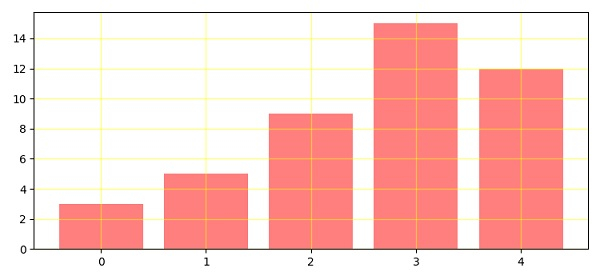# How to draw grid lines behind Matplotlib bar graph?

To draw grid lines behind matplotlib bar graph, we can take the following Steps −

• Make a list of numbers, i.e., data.

• Make a bar using the bar() method, by passing data, color='red' and alpha = 0.5. The alpha blending value should be between 0 (transparent) and 1 (opaque).

• To configure the grid lines, use the grid() method, with color='yellow', linewidth=1, axis='both' and alpha=0.5.

• To display the figure, show() method.

## Example

from matplotlib import pyplot as plt
plt.rcParams["figure.figsize"] = [7.50, 3.50]
plt.rcParams["figure.autolayout"] = True
data = [3, 5, 9, 15, 12]
plt.bar(range(len(data)), data, color='red', alpha=0.5)
plt.grid(color='yellow', linewidth=1, axis='both', alpha=0.5)
plt.show()

## Output